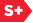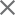### Proceedings Paper

Riesz wavelets and multiresolution structures
Author(s): David R. Larson; Wai-Shing Tang; Eric Weber
Format Member Price Non-Member Price
PDF \$17.00 \$21.00

Paper Abstract

Multiresolution structures are important in applications, but they are also useful for analyzing properties of associated wavelets. Given a nonorthogonal (multi-) wavelet in a Hilbert space, we construct a core subspace. Subsequently, the dilates of the core subspace defines a ladder of nested subspaces. Of fundamental importance are two questions: 1) when is the core subspace shift invariant; and if yes, then 2) when is the core subspace generated by shifts of a single vector, i.e. there exists a scaling vector. If the wavelet generates a Riesz basis then the answer to question 1) is yes if and only if the wavelet is a biorthogonal wavelet. Additionally, if the wavelet generates a tight frame of arbitrary frame constant, then the core subspace is shift invariant. Question 1) is still open in case the wavelet generates a non-tight frame. We also present some known results to question 2) and provide some preliminary improvements. Our analysis here arises from investigating the dimension function and the multiplicity function of a wavelet. These two functions agree if the wavelet is orthogonal. Finally, we discuss how these questions are important for considering linear perturbation of wavelets. Utilizing the idea of the local commutant of a unitary system developed by Dai and Larson, we show that nearly all linear perturbations of two orthonormal wavelets form a Riesz wavelet. If in fact these wavelets correspond to a von Neumann algebra in the local commutant of a base wavelet, then the interpolated wavelet is biorthogonal. Moreover, we demonstrate that in this case the interpolated wavelets have a scaling vector if the base wavelet has a scaling vector.

Paper Details

Date Published: 5 December 2001
PDF: 9 pages
Proc. SPIE 4478, Wavelets: Applications in Signal and Image Processing IX, (5 December 2001); doi: 10.1117/12.449711
Show Author Affiliations
David R. Larson, Texas A&M Univ. (United States)
Wai-Shing Tang, National Univ. of Singapore (Singapore)
Eric Weber, Texas A&M Univ. (United States)

Published in SPIE Proceedings Vol. 4478:
Wavelets: Applications in Signal and Image Processing IX
Andrew F. Laine; Michael A. Unser; Akram Aldroubi, Editor(s)